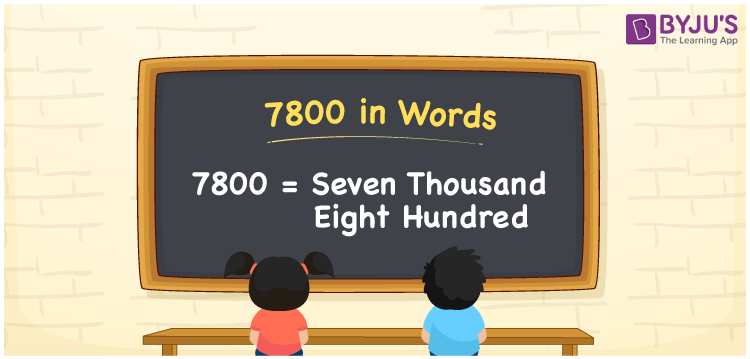# 7800 in Words

7800 in words is “Seven Thousand Eight Hundred”. As we know, 7800 is a cardinal number that represents the value or the count of the object. The number 7800 can be easily converted into words using the place value table. If the price of a table is Rs. 7800, we can write it as “The price of the table is Rs. Seven thousand eight hundred”. Here, we have discussed the procedure for writing the number 7800 in words with a complete explanation.

 7800 in Words: Seven Thousand Eight Hundred. Seven Thousand Eight Hundred in Numerical Form: 7800.

## 7800 in English Words

The number 7800 in English words is given as follows:## How to Write 7800 in Words?

Using the place value system, the number 7800 can be written in words. Below is the place value table for the number 7800.

 Thousands Hundreds Tens Ones 7 8 0 0

The expanded form of the number 7800 is as follows:

= 7 × Thousand + 8 × Hundred + 0 × Ten + 0 × One

= 7 × 1000 + 8 × 100

= 7000 + 800

= 7800

= Seven thousand eight hundred

Hence, 7800 in words is Seven thousand eight hundred.

The number 7800 is a natural number that is succeeded by 7799 and preceded by 7801. Learn more about the number 7800 below:

7800 in words – Seven thousand eight hundred

Is 7800 an odd number? – No

Is 7800 an even number? – Yes

Is 7800 a perfect square number? – No

Is 7800 a perfect cube number? – No

Is 7800 a prime number? – No

Is 7800 a composite number? – Yes

## Frequently Asked Questions on 7800 in Words

Q1

### How to write 7800 in words?

7800 in words is seven thousand eight hundred.

Q2

### Simplify 7000 + 800, and express in words.

Simplifying 7000 + 800, we get 7800. Hence, 7800 in words is seven thousand eight hundred.

Q3

### How to write seven thousand eight hundred in numbers?

Seven thousand eight hundred in numbers is 7800.